# Community posts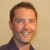by Paul Denny
The University of Auckland

If you have viewed one of your courses in the last day or so you may have noticed a small addition to the main menu. A new score, called the "answer score", now appears (see the screenshot on the right).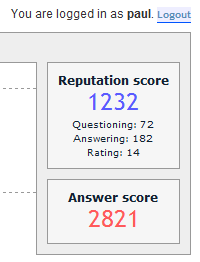The original score and associated algorithm remains unchanged except it has now been renamed to "reputation score" which more accurately reflects its purpose. A number of instructors have been using this original score to assign extra credit to their students - as outlined in the following blog post which also describes the algorithm in detail:

However, this "reputation score" was the source of some confusion for those students who did not read the description of how it was calculated (this description appears if the mouse is hovered over the score area). This is exemplified by the following comment submitted via the PeerWise feedback form:

This is a great tool. I love it. The only criticism is the slow update on the score. You need to wait 30min+ to see what score you have after a session.

This confusion is related to the fact that the "reputation score" for an individual student only increases when other students participate as well (and indicate that the student's contributions have been valued). On the other hand, the new "answer score" updates immediately when a student answers a question and this immediate feedback may alleviate some of the concerns and provide students with another form of friendly competition. As soon as an answer is selected, the number of points earned (or in some cases lost) is displayed immediately as shown in the screenshots below.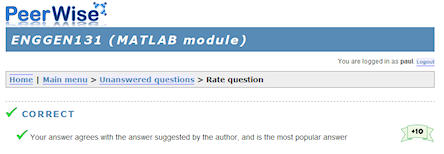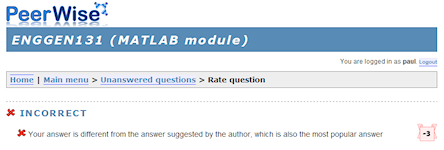More importantly, the new "answer score" now provides another measure of student participation within PeerWise. Instructors may like to use this to set targets for students to reach. A detailed description of how this works follows, but very basically for most students the "answer score" will be close to 10 multiplied by the number of questions they have answered "correctly" (where a correct answer is one that either matches the question author's suggested answer or is the most popular answer selected by peers). For example, the chart below plots the number of answers submitted that agreed with the author's answer (note, this is a lower bound on the number of "correct" answers as just defined previously) against the new "answer score" for all students in one course who were active in the 24 hours after the new score was released. The line is almost perfectly straight and has a slope close to 10.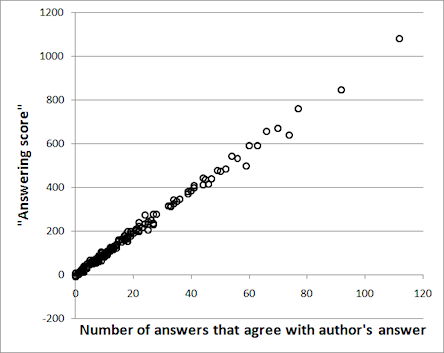A few students fall marginally below the imaginary line with a slope of 10 - this is because every "correct" answer submitted earns a maximum of 10 points however a small number of points are lost if an "incorrect" answer is submitted. The number of points deducted for an incorrect answer depends on the number of alternative answers associated with the multiple-choice question - for example questions with 5 options have a lower associated penalty than questions with 2 options. If a large number of questions are answered by randomly selecting answers (which is obviously a behaviour that we would want to discourage students from adopting), the "answer score" should generally not increase.

So, how can you now begin to make use of this?

It appears to be quite common, from hearing a range of instructors describe how they implement PeerWise in their own classrooms, to require students to answer a minimum number of questions per term. To make things a little more interesting, you can now set an "answer score" target instead. This is practically the same thing but a bit more fun (just remember to multiply by 10) - if you normally ask your students to answer 50 questions, try setting an "answer score" target of 500!